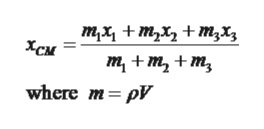# Three cubes, of side L0,2L0, and 3L0, are placed next to one another (in contact) with their centers along a straight line. What is the position, along the line x, of the CM of this system? Assume the cubes are made of the same uniform material and that xCMis measured from the left edge of the smallest cube.Express your answer in terms of L0.

Question
225 views

Three cubes, of side L0,2L0, and 3L0, are placed next to one another (in contact) with their centers along a straight line.

What is the position, along the line x, of the CM of this system? Assume the cubes are made of the same uniform material and that xCM
is measured from the left edge of the smallest cube.

check_circle

Step 1

Given,

Three cubes L0, 2L0, 3L0 are placed next to one another and their centre along a straight line.

Step 2

Schematic diagram for such system has been shown below,

Step 3

Centre of mass for three cube sys...help_outlineImage Transcriptioncloseтx + m,x, + т,х, Хсм т, + m, + m, where m pV fullscreen

### Want to see the full answer?

See Solution

#### Want to see this answer and more?

Solutions are written by subject experts who are available 24/7. Questions are typically answered within 1 hour.*

See Solution
*Response times may vary by subject and question.
Tagged in

### Physics